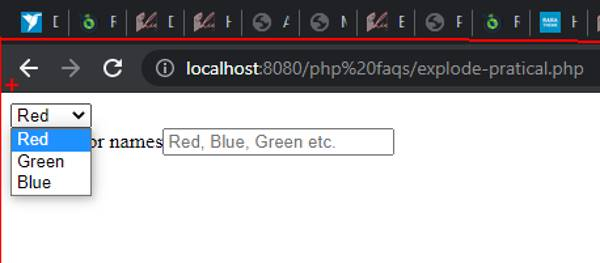Providing Training Since 2009
• Home -
• What is explode function in PHP ? Lets check with practical examples

# What is explode function in PHP ? Lets check with practical examplesIn our PHP training institute in Kolkata students often ask what is explode function and how we can use it.

I will show you why to uses explode function and where to use it. And how to use it practically.

Explode is a PHP function which is used to convert to string into array. The important point here is that the string must be uniformly delimiter. It can be comma, it can be space or it can be any special character.

Example:

\$names =  ‘Rajesh,karim,Mariyam,Meera’;

In the above example, you can see that there is a string called \$names which contains 4 names. The delimiter between names is comma separated.

This is also a valid string for explode

\$colors =  ‘Red Blue Green Yellow’;

In the above case you can see the delimiter here is space and not any special character.

Now lets apply explode function to convert the string into array.

<?php

\$names = ‘Rajesh,karim,Mariyam,Meera’;

\$name_list =  explode(‘,’,\$names);

?>

In the above syntax you can see that we have passed two parameters inside function called explode. The first parameter is the delimiter that the string contains and the second parameter is the name of the string.

So, when we will output the string it will show like this.

<?php

\$names = ‘Rajesh,karim,Mariyam,Meera’;

\$name_list =  explode(‘,’,\$names);

//to print the array

print_r(\$name_list);

?>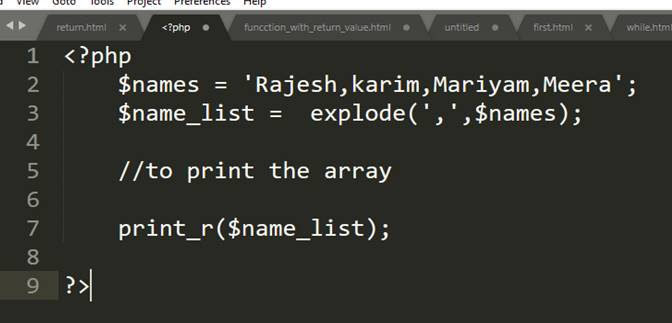Output: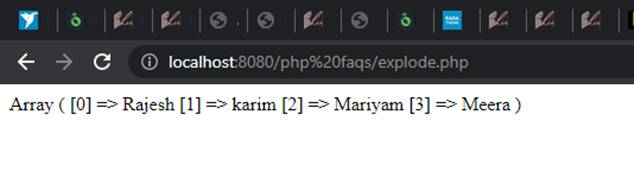In the above output you can see that the string is now converted into array and each name has now  its specific index.

Now suppose you want  to show the name called ‘Meera’ in that case you will have to write

<?php

\$names = ‘Rajesh,karim,Mariyam,Meera’;
\$name_list =  explode(‘,’,\$names);
//to print the array
// print_r(\$name_list);
echo \$name_list;
?>

Output :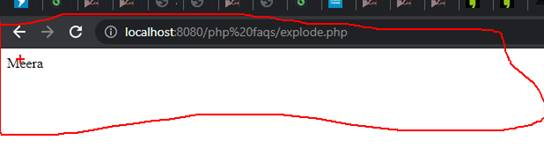As long as the list is small, it’s easier to print one by one. But if the list is very long in that case its not possible to print the names one by one.

In that case we will  use any loop. And the best one will be for loop for showing  the list.

Here is example how you can show the list using for loop.

<?php

\$names = ‘Rajesh,karim,Mariyam,Meera’;

\$name_list =  explode(‘,’,\$names);

//to print the array

// print_r(\$name_list);

//echo \$name_list;

for (\$i=0; \$i <count(\$name_list) ; \$i++) {

echo \$name_list[\$i];

}

?>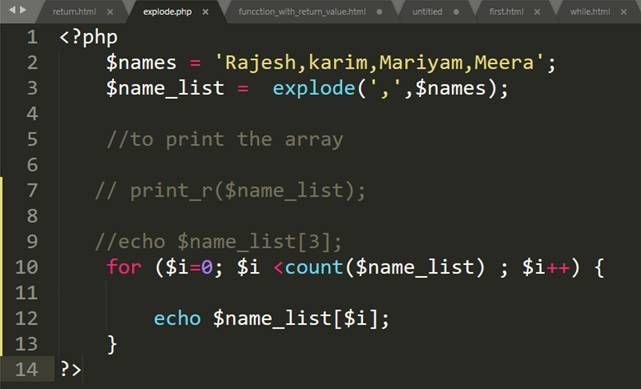Output: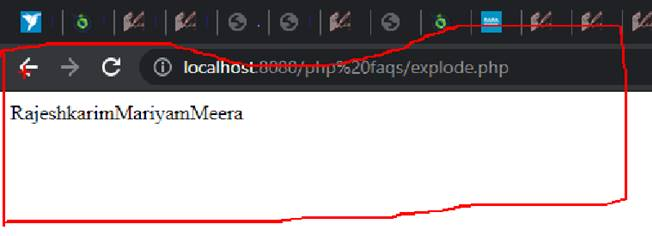If you want to show the names vertically, you can just put break and then it will show the names vertically.

<?php

\$names = ‘Rajesh,karim,Mariyam,Meera’;
\$name_list =  explode(‘,’,\$names);

//to print the array

// print_r(\$name_list);

//echo \$name_list;

for (\$i=0; \$i <count(\$name_list) ; \$i++) {

echo \$name_list[\$i].'<br>’;

}

?>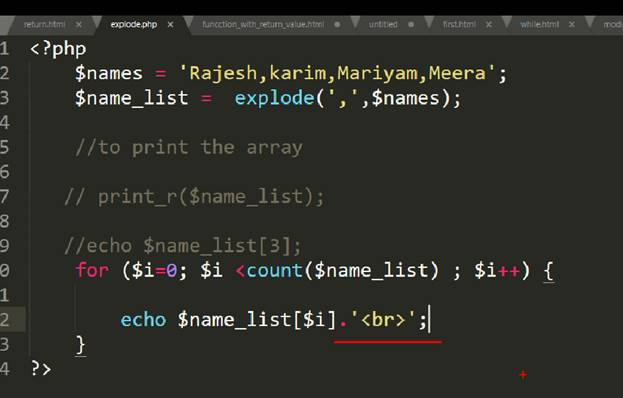Output :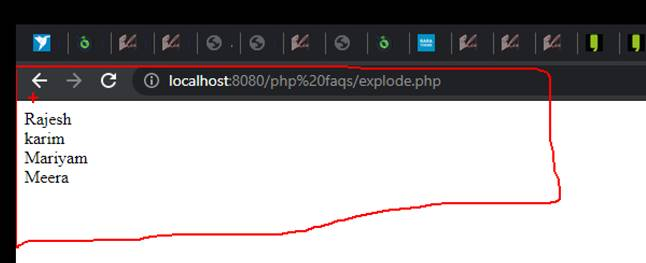Now if you want to show the name list in dropdown, that’s also possible.
Lets now the code to show the names in the dropdown list.

<?php

\$names = ‘Rajesh,karim,Mariyam,Meera’;

\$name_list =  explode(‘,’,\$names);

//to print the array

// print_r(\$name_list);

//echo \$name_list;

echo “<select>”;

for (\$i=0; \$i <count(\$name_list) ; \$i++) {

echo ‘<option value=”‘.\$name_list[\$i].'”>’.\$name_list[\$i].'</option>’;

}

echo “</select>”;

?>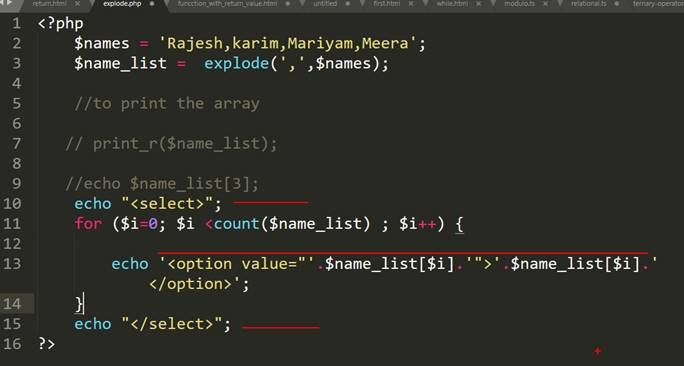Output:Now let’s understand its practical use:

Suppose you are developing an e commerce website and in admin side you want  your customer to enter color names.

In that case you can ask the admin to enter comma separated color names and in front side you can show the list of the color to select by the uses.

Let’s see with example.

<?php

if(\$_POST){

\$color_names = \$_POST[‘color’];

// save this into database

// fetch from database in the front section

// this is front part

\$colors= explode(‘,’,\$color_names);

echo ‘<select>’ ;

for(\$i=0;\$i<count(\$colors);\$i++){

// use here while loop for fetching the data

echo ‘<option value=”‘.\$colors[\$i].'”>’.\$colors[\$i].'</option>’;

}

echo ‘</select>’ ;

}

?>

<form method=”post” action=””>

Enter color names<input type=”text” name=”color” placeholder=”Red, Blue, Green etc.”><br>

<input type=”submit” name=”submit” value=”Submit”>

</form>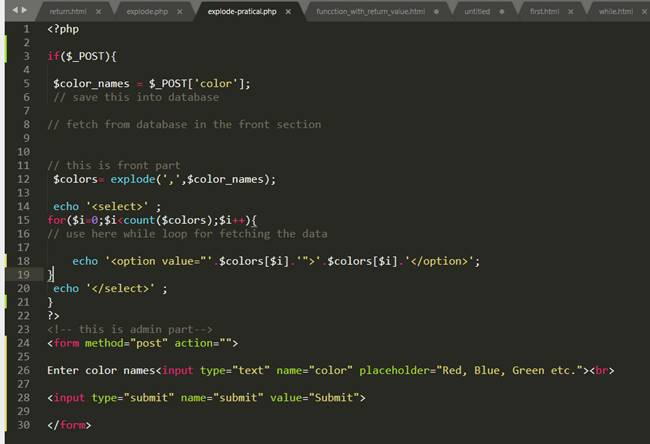Output: